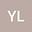•In this paper, we considered the global regularity for the 2D incompressible anisotropic magnetic B\’{e}nard system with fractional partial dissipation. More precisely, we established the global existence and regularity for the 2D incompressible anisotropic magnetic B\’{e}nard system with only vertical hyperdiffusion $\Lambda_{2}^{2\beta}b_1$ and horizontal hyperdiffusion $\Lambda_{1}^{2\beta}b_2$ and $(-\Delta)^{\alpha}\theta$, where $\Lambda_{1}$ and $\Lambda_{2}$ are directional Fourier multiplier operators with the symbols being $|\xi_1|$ and $|\xi_2|$, respectively. We prove that, for $\beta>1$ and $0<\alpha<1$, this system always possesses a unique global-in-time classical solution when the initial data is sufficiently smooth.